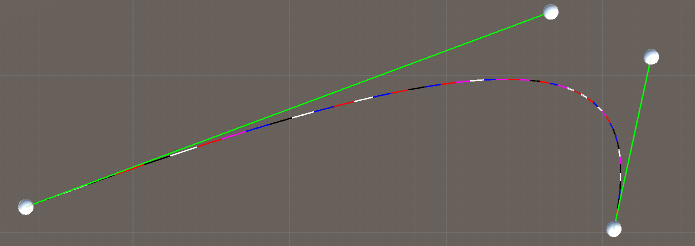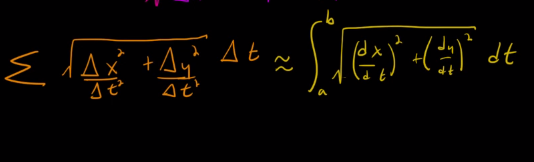# Everything about interpolation in Unity with C# code

## 3. Move along a Bezier curve

The focus here is on the Bezier curve. But the ideas are the same if you want to move along a Catmull-Rom spline. The problem we want to solve is that the parameter t we have used to determine where we are on a spline is NOT always percentage of distance along the line. The reason is that the distances between each t step may be different depending on the positions of the points that determines the shape of the spline. As you can see in the image below where I've interpolated the Bezier curve with constant t steps, the individual line segments don't have the same length:If you have a camera which need to move along the curve with constant steps you need to modify the curve to make sure each step has the same size. It's by the way common to move cameras along cubic splines. And yes, you could move the camera with constant speed (with something like Transform.Translate(Vector3.forward * speed * Time.deltatime)) and rotate it towards waypoints along the curve, but maybe that's not always possible. The solution to the problem is that we have to reparametrize the curve to make each step the same size.

To make each step the same size you need to learn a few things. Luckily, people have already made detailed explanations on YouTube, so there's no need to flood the Internet with more of the same information. So if you are interested in understanding what's going on here, you should watch the following (or if you aldready have the knowledge needed, or if you are just interested in the code, you can ignore them):

To sum it up, the basic idea is that we have to first learn how to compute the length of the Bezier curve, and then how to compute where we are on a curve at certain positions. We already know how to compute where we are on a curve based on the parameter t. But now we imagine that we move along a straight line at constant steps, and at each step we have to translate the straight line to a position on the curve by calculating the curve's t value (to get a position on the curve) as if we had traveled along a curve.

### Calculate the length of the curve

To be able to compute the length of a curve we will use two methods. Both of these methods involve a root (which is not good from a performance perspective), so I'm not sure which one is the fastest, but one of them is most likely more accurate than the other.

#### Method 1. Straight lines

Here we loop through the Bezier curve in the same way as when we created it. But we will also calculate the length of each section and add them up to get the total length. So we will approximate the Bezier curve with a lot of small straight lines. This is the code:

```//Get the length of the curve with a naive method where we divide the
//curve into straight lines and then measure the length of each line
float GetLengthNaive(float tStart, float tEnd)
{
//This is the resolution, the higher the better
int sections = 10;

//Divide the curve into sections
float delta = (tEnd - tStart) / (float)sections;

//The start position of the curve
Vector3 lastPos = DeCasteljausAlgorithm(tStart);

//Init length
float length = 0f;

//Move along the curve
for (int i = 1; i <= sections; i++)
{
//Calculate the t value at this section
float t = tStart + delta * i;

//Find the coordinates at this t
Vector3 pos = DeCasteljausAlgorithm(t);

//Add the section to the total length
length += Vector3.Magnitude(pos - lastPos);

//Save the latest pos for next loop
lastPos = pos;
}

return length;
}
```

#### Method 2. Simpson's Rule

Here we loop through the Bezier curve in the same way as when we created it. But instead of approximating each section with a straight line, we will approximate each section with a second degree polynomial. The easiest way to do that is to use Simpson's rule.

To be able to use Simpson's rule we need an integral that calculates the length of the line. Luckily, the nice people in the YouTube videos gave us that integral (but we have three dimensions, so just add dz to it):It's not possible to find an analytic solution to that integral, which is why we have to approximate it with Simpson's rule. But first we need to figure out what's inside the integral. If we have a Bezier curve, what's inside the integral is the derivative of the Bezier curve with respect to t. So you have to take the derivative of De Casteljau's Algorithm. This is easy: you just combine all different layers into one big equation called U, simplify, and then take the derivative. The result is this:

```//The derivative of cubic De Casteljau's Algorithm
Vector3 DeCasteljausAlgorithmDerivative(float t)
{
Vector3 dU = t * t * (-3f * (A - 3f * (B - C) - D));

dU += t * (6f * (A - 2f * B + C));

dU += -3f * (A - B);

return dU;
}
```

But what's inside the integral is actually the magnitude of the derivative, so we need another method:

```//Get and infinite small length from the derivative of the curve at position t
float GetArcLengthIntegrand(float t)
{
//The derivative at this point (the velocity vector)
Vector3 dPos = DeCasteljausAlgorithmDerivative(t);

//This the how it looks like in the YouTube videos
//float xx = dPos.x * dPos.x;
//float yy = dPos.y * dPos.y;
//float zz = dPos.z * dPos.z;

//float integrand = Mathf.Sqrt(xx + yy + zz);

//Same as above
float integrand = dPos.magnitude;

return integrand;
}
```

And now we can use Simpson's rule to approximate the integral to get the length of the Bezier curve between two t values:

```//Get the length of the curve between two t values with Simpson's rule
float GetLengthSimpsons(float tStart, float tEnd)
{
//This is the resolution and has to be even
int n = 20;

//Now we need to divide the curve into sections
float delta = (tEnd - tStart) / (float)n;

//The main loop to calculate the length

//Everything multiplied by 1
float endPoints = GetArcLengthIntegrand(tStart) + GetArcLengthIntegrand(tEnd);

//Everything multiplied by 4
float x4 = 0f;
for (int i = 1; i < n; i += 2)
{
float t = tStart + delta * i;

x4 += GetArcLengthIntegrand(t);
}

//Everything multiplied by 2
float x2 = 0f;
for (int i = 2; i < n; i += 2)
{
float t = tStart + delta * i;

x2 += GetArcLengthIntegrand(t);
}

//The final length
float length = (delta / 3f) * (endPoints + 4f* x4 + 2f * x2);

return length;
}
```

So which of the methods is most accurate? The answer is that we don't know because we don't know the exact length of the line. But if you test the methods you get the following:

• Small straight lines: 860.0575 m
• Simpson's rule: 861.2954 m

...so the difference between the methods is not big.

### Divide the curve into constant steps

Alright, finding the length of the Bezier curve was cool, but why do we need it? Well, if you have watched the YouTube videos you know that we need it to solve this equation:The above equation answers the question: at which t value have we walked 1/3 of the total length of the curve? In the future we will replace this 1/3 with however far we have walked on the line. This distance is always measured from the start of the line. To solve the equation we are going to use a numerical method called Newton's method. The basic idea behind the method is that it starts with a t value, and then it's trying to find a better t value by using the t value from the last iteration. This is how it looks like in code:

```//Use Newton–Raphsons method to find the t value at the end of this distance d
float FindTValue(float d, float totalLength)
{
//Need a start value to make the method start
//Should obviously be between 0 and 1
//We can say that a good starting point is the percentage of distance traveled
//If this start value is not working you can use the Bisection Method to find a start value
//https://en.wikipedia.org/wiki/Bisection_method
float t = d / totalLength;

//Need an error so we know when to stop the iteration
float error = 0.001f;

//We also need to avoid infinite loops
int iterations = 0;

while (true)
{
//Newton's method
float tNext = t - ((GetLengthSimpsons(0f, t) - d) / GetArcLengthIntegrand(t));

//Have we reached the desired accuracy?
if (Mathf.Abs(tNext - t) < error)
{
break;
}

t = tNext;

iterations += 1;

if (iterations > 1000)
{
break;
}
}

return t;
}
```

To be able to better see the line sections, I've added a way to display a new color each new step. To make that work you need to add this to the top of the script:

```//An array with colors to display the line segments that make up the final curve
Color[] colorsArray = {Color.white, Color.red, Color.blue, Color.magenta, Color.black};
```

And finally the method that will divide the Bezier curve into equal steps:

```//Divide the curve into equal steps
void DivideCurveIntoSteps()
{
//Find the total length of the curve
float totalLength = GetLengthSimpsons(0f, 1f);

//How many sections do we want to divide the curve into
int parts = 10;

//What's the length of one section?
float sectionLength = totalLength / (float)parts;

//Init the variables we need in the loop
float currentDistance = 0f + sectionLength;

//The curve's start position
Vector3 lastPos = A;

//The Bezier curve's color
//Need a seed or the line will constantly change color
Random.InitState(12345);

int lastRandom = Random.Range(0, colorsArray.Length);

for (int i = 1; i <= parts; i++)
{
//Use Newton–Raphsons method to find the t value from the start of the curve
//to the end of the distance we have
float t = FindTValue(currentDistance, totalLength);

//Get the coordinate on the Bezier curve at this t value
Vector3 pos = DeCasteljausAlgorithm(t);

//Draw the line with a random color
int newRandom = Random.Range(0, colorsArray.Length);

//Get a different random number each time
while (newRandom == lastRandom)
{
newRandom = Random.Range(0, colorsArray.Length);
}

lastRandom = newRandom;

Gizmos.color = colorsArray[newRandom];

Gizmos.DrawLine(lastPos, pos);

//Save the last position
lastPos = pos;

//Add to the distance traveled on the line so far
currentDistance += sectionLength;
}
}
```

Now we can compare if all this math was really worth it:If you can't tell the difference then you've wasted a lot of time, but maybe learned something new! Otherwise you've just learned how to take constant steps along a Bezier curve!